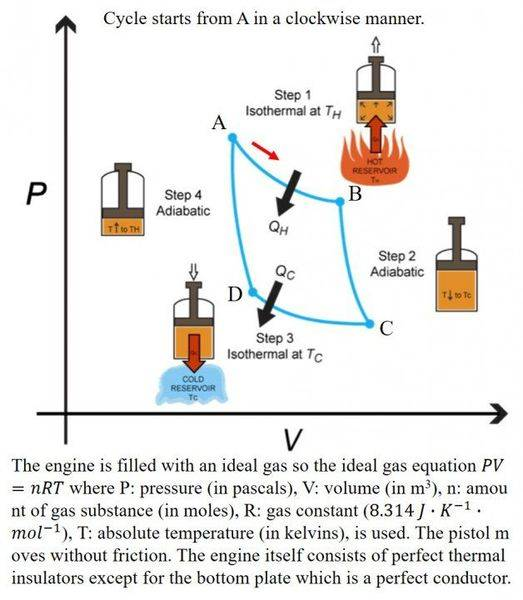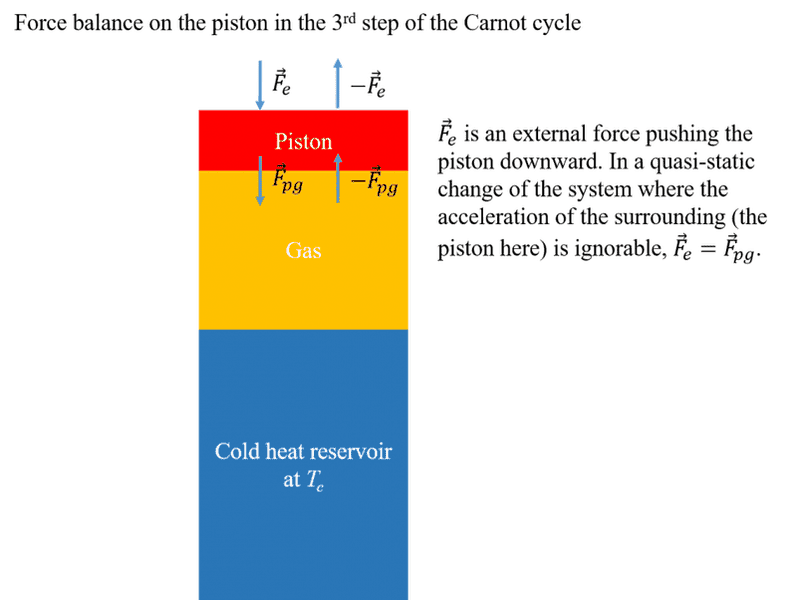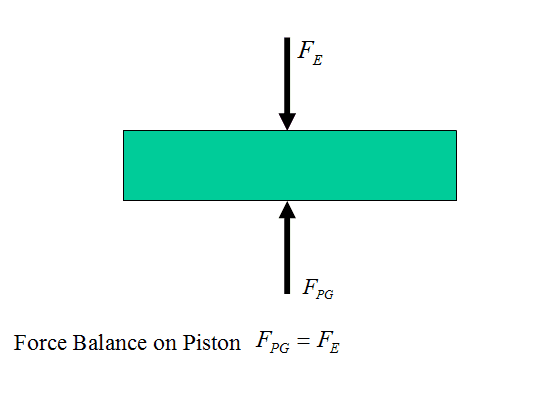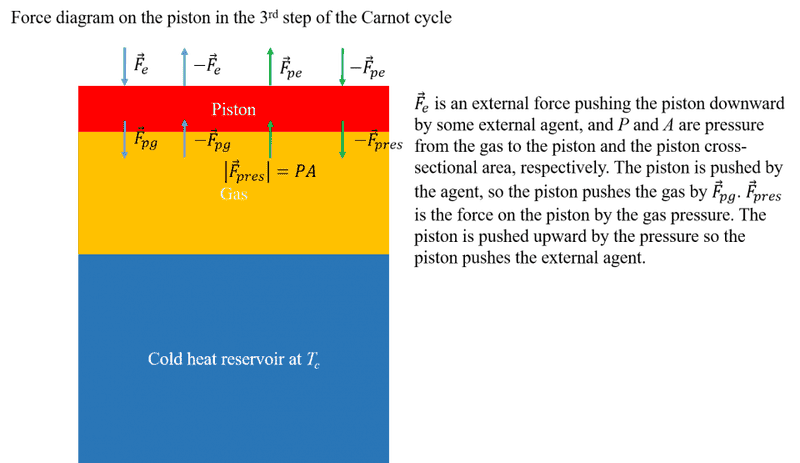In 3rd step of Carnot cycle, work done on the piston is pdV?

Hello.

First, look at the figure describing Carnot's cycle.In 1st step (A → B) and 2nd step (B → C), I fully understand that the work done on the pistol (surrounding) by the gas (system) is dW = pdV where dV = Adl since F = pA is the only force on the pistol from the gas (I assumed there are no other forces). So far so good. However, in 3rd step (C → D) I have a confusion about the work on the pistol by the gas. In this step, external force Fe applies on the pistol downward direction to compress the gas, so eventually, Fe applies on the gas from the pistol. And according to Newton's 3rd law (action-reaction law), the gas also applies the force -Fe on the pistol. As a result, the gas applies not only the force associated with the gas pressure (Fp = pA), but also -Fe . So. I think the work done on the piston by the gas must be (-Fe + Fp)dl, instead of only Fpdl = pdV.

Am I wrong? If it is, could you please point out exactly what I am wrong with?

Thanks for reading this post and I'm happily waiting for your response.

If you are considering the third law.
You should be saying the external force is exerting on the piston and so the piston will exert an opposite force on the force giver.
The gas and the external force are separated by a wall.

Chestermiller
Mentor
In considering Newton's 3rd law, the force that A exerts on B is equal and opposite to the force that B exerts on A. But, for either body, we don't say that there is no net force. In the force balance for A, we only include the force exerted by B on A, and in the force balance on B, we only include the force exerted by A on B. We don't include both. In considering the work done on A, we only include the work done by the force exerted by B on A, and in considering the work done on B, we only include the work done by the force exerted by A on B.

•goodphy
Philip Wood
Gold Member
Your –Fe is the force exerted by the gas. It's the gas that resists the pushing in of the piston. I suppose we really ought to consider the piston itself, so…
Fe is N3l partner force exerted by piston on external agency that exerts Fe on piston
Fe is N3l partner force exerted by gas on piston that exerts Fe on gas
In this analysis there are two forces on the piston, one from the gas and one from the external agency. They are equal and opposite, not because they are a N3L pair (they aren't!) but because the pushing in of the piston is quasi-static.

•goodphy
Your –Fe is the force exerted by the gas. It's the gas that resists the pushing in of the piston. I suppose we really ought to consider the piston itself, so…
Fe is N3l partner force exerted by piston on external agency that exerts Fe on piston
Fe is N3l partner force exerted by gas on piston that exerts Fe on gas
In this analysis there are two forces on the piston, one from the gas and one from the external agency. They are equal and opposite, not because they are a N3L pair (they aren't!) but because the pushing in of the piston is quasi-static.

Hello.

I think you suggested me a way to break through my confusion. Yes, the piston is receiving forces from two things simultaneously; one is the external agency pushing the piston by the force Fe, and another one is the gas pushing the piston due to Newton's 3rd law Fgp (external agency pushes the piston → the piston pushes the gas → the gas pushes the piston according to the Newton's 3rd law ). If Fgp = - Fe , then a sum of these forces is zero so work done on the piston by the agency is zero (Fgp is also essentially from the agency).

Can I have a further question? At this moment, I'm not 100 % convinced that Fgp = - Fe . I'm not able to clear prove this. I think you mentioned "quasi-static" to justify this relation. Could you please tell me how "quasi-static" is related to Fgp = - Fe ? What does "quasi-static" actually mean?

Chestermiller
Mentor
Let's go back to freshman physics. Take the piston as a free body, and write a Newton's 2nd law force balance on the piston. What do you get?

•goodphy
Philip Wood
Gold Member
I don't want to seem patronising, but I'd like to congratulate you on the precision with which you are phrasing your questions.

A quasi-static change is one that is so slow that the system is always infinitesimally near a state of thermodynamic equilibrium. [I took this from M W Zemansky: Heat and Thermodynamics. It needs illustrating with examples, which a good textbook will do. However, one direct consequence is the absence of finite accelerations of the piston or of regions of the gas. Now go back to Chestermiller's comment!

I think you've probably go a good understanding of Newton's third law, but one common misconception is to think of body A pushing body B, and B responding by pushing A. One force does not cause the other to arise; rather we have a 'force-pair' symmetrical in its operation.

•goodphy
I see.

I think I finally got the right answer. Thanks for all of you to guide me along a right track!

I attached the summary of what I've learned from your answers and I hope this would helpful for other guys who have similar question.Chestermiller
Mentor
Suppose the acceleration of the piston is not negligible. What is the force balance equation then?

Chestermiller
Mentor
I see.

I think I finally got the right answer. Thanks for all of you to guide me along a right track!

I attached the summary of what I've learned from your answers and I hope this would helpful for other guys who have similar question.

View attachment 114605
The arrow on Fpg is pointing in the wrong direction, if it represents the force that the gas exerts on the piston.

Chestermiller
Mentor
Here is the proper force balance on the piston (assumed massless and frictionless):So, the differential work done by the gas on the piston is:
$$dW=F_{PG}dx=F_Edx$$where dx is the differential upward displacement of the piston. If dx is negative, it means that the displacement of the piston is downward, and the work done by the gas on the piston is negative (i.e., the piston does work on the gas). The change in volume is given by dV=Adx. Therefore, $$dW=F_{PG}\frac{dV}{A}=P_{PG}dV=F_E\frac{dV}{A}=P_EdV$$So, in summary,$$dW=P_{PG}dV=P_EdV$$It's as simple as that.

•goodphy
Here is the proper force balance on the piston (assumed massless and frictionless):
View attachment 114665

I would like to simply accept your force balancing on the pistol; your drawing really fits what the thermodynamic textbook try to explain. However, I'm still in trouble. Let's have an extension of your drawing.Here, there is another force on the pistol from the gas, which is -Fpg. The piston is pushed by Fe so the piston pushes the gas by Fpg . The situation is reminiscent to that a person A pushes his friend B leaning on a soft wall; A pushes B so B pushes the wall. As a result, there is the reaction force to Fpg, which is -Fpg.

Could you tell me why this force is not included in the calculation of work on the piston?

Philip Wood
Gold Member
Didn't you take on board the first sentence of my original post (number 4)? Looking on the diagram at the row of four arrows at the piston-gas interface, -Fpg is the same thing as Fpress; you're showing the same force twice! Similarly with the downward arrows; there's only one force: you're showing it twice.

Chestermiller
Mentor

I would like to simply accept your force balancing on the pistol; your drawing really fits what the thermodynamic textbook try to explain. However, I'm still in trouble. Let's have an extension of your drawing.

View attachment 114695

Here, there is another force on the pistol from the gas, which is -Fpg. The piston is pushed by Fe so the piston pushes the gas by Fpg . The situation is reminiscent to that a person A pushes his friend B leaning on a soft wall; A pushes B so B pushes the wall. As a result, there is the reaction force to Fpg, which is -Fpg.

Could you tell me why this force is not included in the calculation of work on the piston?
When you do a force balance on an object, you only include in the free body diagram the forces that other objects exert on it. You don't include the forces that it exerts on the other objects. And you only include the forces acting by direct contact.

In my diagram, the gas exerts a force ##F_{pg}## on the piston (and this is included in the force balance on the piston). Of course, the piston exerts and equal and opposite force of ##-F_{pg}## on the gas, but this is not included in the force balance on the piston; it is included in the force balance on the gas. Similarly, the external agent exerts a force of ##F_E## on the piston (and this is included in the force balance on the piston). Of course, the piston exerts and equal and opposite force of ##-F_E## on the external agent, but this is not included in the force balance on the piston; it is included in the force balance on the external agent.

When you do a free body diagram, you only include the forces acting on the body by direct contact.

If you want to see how this works for A, B, and the Wall, it goes like this:

##F_{GA}## = force exerted by the ground on A in + x direction
##F_{AB}## = force exerted by A on B in + x direction
##-F_{AB}##=force exerted by B on A in + x direction
##F_{BW}##=force exerted by B on Wall in + x direction
##-F_{BW}##= force exerted by Wall on B in + x direction
##F_WG##=force exerted by Wall on Ground in + x direction
##-F_{WG}##=force exerted by ground on Wall in + x direction

Force balance on A: ##F_{GA}-F_{AB}=0##

Force balance on B: ##F_{AB}-F_{BW}=0##

Force balance on Wall: ##F_{BW}-F_{GW}=0##

Last edited:
•goodphy
Looking on the diagram at the row of four arrows at the piston-gas interface, -Fpg is the same thing as Fpress; you're showing the same force twice! Similarly with the downward arrows; there's only one force: you're showing it twice.

Hello.

I really don't want you to be bothered; But..why is -Fpg same to Fpres ? I think they're different entities. Fpres is from the collisions of gas particles on the piston bottom wall, while Fpg is from the state that the piston is pushing the gas downward. Their origins are different, so they're different ones, I think.

Can you tell me why they're same?

Philip Wood
Gold Member
If, in an inertial frame of reference, a body experiences a force, then that force must be exerted on it by another thing. Your Fpres on the piston is a good example – the force on the piston is exerted by the gas molecules. But what external thing, are you supposing, exerts –Fpg ? I'm afraid that "from the state that the piston is pushing the gas downward" doesn't answer this question. If you can't pin down the external thing, then the force doesn't exist – or (as in this case) you are just re-naming a force that does exist (Fpres).

[When you say "their origins are different", your interpretation of 'origins' is not a useful one. For 'origins', read 'external bodies exerting the force' and you do now have a useful interpretation. In this case, of course, the origins are the same, because only one body exerts an upward force on the bottom of the piston, namely the gas. Note that this force would have almost the same value, for a given gas volume, if the piston weren't moving.]

Last edited:
If, in an inertial frame of reference, a body experiences a force, then that force must be exerted on it by another thing. Your Fpres on the piston is a good example – the force on the piston is exerted by the gas molecules. But what external thing, are you supposing, exerts –Fpg ? I'm afraid that " from the state that the piston is pushing the gas downward" doesn't answer this question. If you can't pin down the external thing, then the force doesn't exist – or (as in this case) you are just re-naming a force that does exist (Fpres).

Hm...I got some idea right now. The force –Fpg may not be taken into account in a calculation of the work done on the piston since the origin of this force is the piston itself; the work with –Fpg on the piston may be the work done by the piston itself via the reaction force. It is reminiscent of the situation that a person A pushes the hard wall. His upper body will be pushed back due to the reaction force from the wall, however, in this case, the wall doesn’t do work on A, no energy is drained from the wall, it is just that A works on himself via the reaction force.

So...there may be a rule that reaction forces must not be included in the calculation of the work. I hope I finally got on a right track.

Philip Wood
Gold Member
The force –Fpg may not be taken into account in a calculation of the work done on the piston since the origin of this force is the piston itself
If –Fpg is a force on the piston, then its origin can't be the piston itself. Please read post 16 carefully, and think about what it means.

Chestermiller
Mentor
@goodphy: You need to go back and review freshman physics. Your posts in this thread have shown that (1) you no longer remember how to draw a proper free body diagram and (2) you no longer remember how to properly apply Newton's 3rd law. These skills are required to correctly do thermodynamics. It doesn't seem possible to help you until you have remastered these skills.

Chet